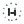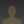0%
0%

# Inductance LC Meter Using Arduino

In this project, we will display Inductance as well as capacitance along with frequency on 16X2 LCD.

Similar projects worth following
545 views
In this project, we calculate inductance and capacitance by employing an LC circuit in parallel. And with the help of 16 x2 LCD, we will display Inductance, capacitance as well as frequency.

First, we estimate the frequency by utilizing Arduino and by using a few mathematical calculations we can determine the inductance or capacitance.

We are going to use an LC circuit frequency response formula.

f=1/(2*time) where time is output of pulseIn() function

then we have LC circuit Frequency:

f=1/2*Pi* (square root of LC)

by solving the above equations we get inductance as follows

L=1/(4* pi* pi* f* f* c)

We have a frequency that can be turned into inductance by utilizing the above formula.

We have used Arduino for further operation. The LC circuit contains an Inductor and a capacitor. We have used an operational amplifier ( 741 ) to convert sinusoidal resonance frequency to a digital or square wave. We have used a 3v battery connected in reverse polarity to get the correct output frequency.

Another we have used the push button to change the operation mode (either we are measuring inductance or capacitance). LCD is used for display purposes.

• 1 × Arduino Uno
• 1 × 741 OPAMP
• 1 × 3V battery
• 1 × 1n4007 diode
• 1 × Resistor 100 ohm
• ### Run a Program

#include
LiquidCrystal lcd(A5, A4, A3, A2, A1, A0);

#define serial

#define charge 3
#define freqIn 2
#define mode 10

#define Delay 15

double frequency, capacitance, inductance;

typedef struct
{
int flag: 1;
}Flag;

Flag Bit;

void setup()
{
#ifdef serial
Serial.begin(9600);
#endif
lcd.begin(16, 2);
pinMode(freqIn, INPUT);
pinMode(charge, OUTPUT);
pinMode(mode, INPUT_PULLUP);
lcd.print(" LC Meter Using ");
lcd.setCursor(0, 1);
lcd.print(" Arduino ");
delay(2000);
lcd.clear();
lcd.print("Circuit Digest");
delay(2000);
}

void loop()
{
for(int i=0;i 0.1)
frequency+= 1.E6 / (2 * Pulse);
delay(20);
}
frequency/=Delay;
#ifdef serial
Serial.print("frequency:");
Serial.print( frequency );
Serial.print(" Hz ");
#endif

lcd.setCursor(0, 0);
lcd.print("freq:");
lcd.print( frequency );
lcd.print(" Hz ");

if (Bit.flag)
{
inductance = 1.E-3;
capacitance = ((1. / (inductance * frequency * frequency * 4.*3.14159 * 3.14159)) * 1.E9);
if((int)capacitance < 0)
capacitance=0;
#ifdef serial
Serial.print("Capacitance:");
Serial.print( capacitance,6);
Serial.println(" uF ");
#endif
lcd.setCursor(0, 1);
lcd.print("Cap: ");
if(capacitance > 47)
{
lcd.print( (capacitance/1000));
lcd.print(" uF ");
}
else
{
lcd.print(capacitance);
lcd.print(" nF ");
}
}

else
{
capacitance = 0.1E-6;
inductance = (1. / (capacitance * frequency * frequency * 4.*3.14159 * 3.14159)) * 1.E6;
#ifdef serial
Serial.print("Ind:");
if(inductance>=1000)
{
Serial.print( inductance/1000 );
Serial.println(" mH");
}
else
{
Serial.print( inductance );
Serial.println(" uH");
}
#endif

lcd.setCursor(0, 1);
lcd.print("Ind:");
if(inductance>=1000)
{
lcd.print( inductance/1000 );
lcd.print(" mH ");
}
else
{
lcd.print( inductance );
lcd.print(" uH ");
}
}

{
Bit.flag = !Bit.flag;
delay(1000);
}
delay(50);
}

Share

## Similar Projects

Project Owner Contributor

### Arduino Capacitance MeterhIOTron

Project Owner Contributor

### Arduino Frequency Generatorprojecter

# Does this project spark your interest?

Become a member to follow this project and never miss any updates